Courses

# Test: Vapour Pressure

## 18 Questions MCQ Test Chemistry for JEE | Test: Vapour Pressure

Description
This mock test of Test: Vapour Pressure for JEE helps you for every JEE entrance exam. This contains 18 Multiple Choice Questions for JEE Test: Vapour Pressure (mcq) to study with solutions a complete question bank. The solved questions answers in this Test: Vapour Pressure quiz give you a good mix of easy questions and tough questions. JEE students definitely take this Test: Vapour Pressure exercise for a better result in the exam. You can find other Test: Vapour Pressure extra questions, long questions & short questions for JEE on EduRev as well by searching above.
QUESTION: 1

### Direction (Q. Nos. 1-9) This section contains 9 multiple choice questions. Each question has four choices (a), (b), (c) and (d), out of which ONLY ONE option is correct. Q. A person living in Shimla observed that cooking of food without using pressure cooker takes more time. The reason for this observation is that at high altitude

Solution:

At high altitude, atmospheric pressure is low. Therefore, liquids at high altitude boil at lower temperatures in comparison to that at sea level hence pressure cooker is used for cooking food.

QUESTION: 2

### Atmospheric pressures recorded in four metro cities are as follows :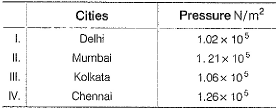Q. Based on the above data, order in which water will boil (starting the earliest) in these cities is

Solution:

For water to boil, it needs to balance the atmospheric pressure exerted on it. It will be easy for water to boil when atmospheric pressure is less. So for the answer, the pressure would be arranged from least pressure to highest pressure and this will be the order of decreasing ease of boiling point.

QUESTION: 3

### At a given temperature, total vapour pressure (in torr) of a mixture of volatile components A and B is g iven by ptotal = 120 - 75 x B. Hence, vapour pressure of pure A and B respectively are

Solution: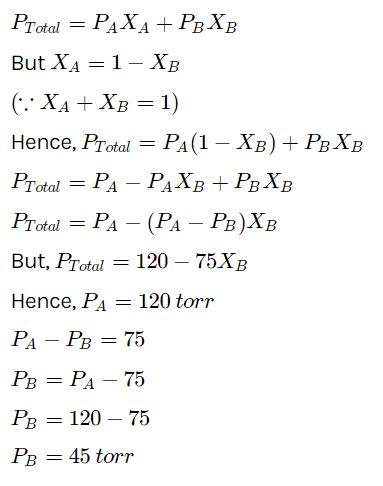QUESTION: 4

Vapour pressure of mixture of 1 mole volatile liquid A and 1 mole volatile liquid B is 350 mm Hg at 50°C. On adding 2 moles of A Into mixture, vapour pressure increases by 25 mm Hg. Thus, vapour pressure of pure components are (in mm Hg)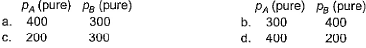Solution:

We have from Raoult’s law;-
Pmixture = xaPa+xbPb
350 = 1/2×Pa+1/2×Pb
Pa+Pb = 700
And 375 = 3/4Pa+1/4pb
On solving both equations, we get Pa = 400 and Pb = 300

QUESTION: 5

Vapour pressure of a liquid is 380 mm Hg at 300 K and 570 mm Hg at 350 K. Thus, its normal boiling point is (log2 = 0.3010, log 1.5= 0.1761)

Solution:
QUESTION: 6

Heat of vaporisation of H2O is 40.6 kJ mol-1. At a 4000 m attitude , the atmospheric pressure is about 0.605 atm. What boiling point would you expect for water under these conditions?

Solution:
QUESTION: 7

Arrange ortho, meta and para-nitrophenols in increasing boiling points

Solution:

Para has max packing efficiency due to its symmetrical structure and it also forms intermolecular H-bonds. Meta derivative has comparatively low packing efficiency but forms intermolecular H-bonds and ortho derivative has the least packing efficiency and does not form intermolecular hydrogen bonds instead it forms intramolecular hydrogen bond which doesn't have any role in increasing the boiling point.

QUESTION: 8

A chemist decides to find the vapour pressure of water by the gas saturation method . 100 L of N2 gas is passed through 65.44 g of water. After passage of the gas, 63.13 g water remained. The temperature of water is 298 K.

Thus, vapour pressure of water is

Solution:
QUESTION: 9

Choose the correct statement with respect to the vapour pressure of a liquid among the following.

Solution:

Vapour pressure is defined as the pressure exerted by the vapours above the liquid surface in equilibrium with the liquid at a given temperature. The vapour pressure of a liquid increases non-linearly with increasing temperature.
This is because kinetic energy is the function of temperature which means that as the temperature is increased, more molecules will have greater kinetic energies and thus they can escape from the surface of the liquid to the vapour phase resulting in higher vapour pressure.

*Multiple options can be correct
QUESTION: 10

Direction (Q. Nos. 10-11) This section contains 4 multiple choice questions. Each question has four choices (a), (b), (c) and (d), out of which ONE or  MORE THANT ONE  is correct.

Q. HF molecule also exist in liquid state. Types of intermolecular forces between them a

Solution: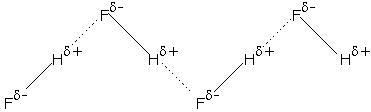Here, both dipole-dipole interaction and intermolecular H-bonding takes place which makes HF a liquid.

*Multiple options can be correct
QUESTION: 11

Clausius-Clapeyron equation can be expressed as

Solution:

Clausius-Clapeyron equation is p = Ae-∆Hvap / RT
Taking ln on both sides, ln p = ln A - ∆Hvap / RT
For two different temperature, we have
ln p1 = ln A - ∆Hvap / RT1 ------------(i)
And ln p2 = ln A - ∆Hvap / RT2---------------(ii)
(activation energy, A remains same even the Temperature changes)
(ii)-(i)
ln p2-ln p1 = ∆Hvap/R [1/T2-1/T1]
Or log p2/p1 = ∆Hvap /2.303 R [1/T2-1/T1]

QUESTION: 12

Direction (Q. Nos. 12-15) This section contains a paragraph, wach describing theory, experiments, data etc. three Questions related to paragraph have been given.Each question have only one correct answer among the four given options (a),(b),(c),(d).

Passage I

For a given liquid at a given temperature vapour pressure is given by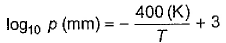Q. Thus, vapour pressure of the liquid at 400 K is

Solution:

Substitute T equal to 400 K in RHS and solve the log.

log(base 10)p = 2

⇒ 10² = p

⇒ p = 100 mm

QUESTION: 13

Passage I

For a given liquid at a given temperature vapour pressure is given byQ. Latent heat of vaporisation in the given temperature range is

Solution:
QUESTION: 14

Passage II

Variation of log10 p with 1/T is given in the following graph (pressure in bar)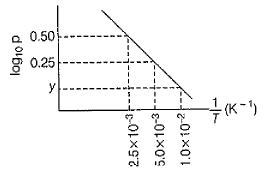Q. Enthalpy of vaporisation based on this data is

Solution:

We have, log p2/p1 = ∆Hvap / 2.303 R [1/T2-1/T1]
Or log p2-log p1 = ∆Hvap / 2.303 R [1/T2-1/T1]
On putting necessary value
0.5-0.25 = ∆Hvap / 2.303 2 [2.5 10-3 - 5 10-3]
∆Hvap=-0.25× 2.303× 2/2.5× 10-3
∆Hvap = -460 cal mol-1

QUESTION: 15

Passage II

Variation of log10 p with 1/T is given in the following graph (pressure in bar)Q. What is value of y, at point 1.0 x 10-2 K-1 on x-axis ?

Solution:
QUESTION: 16

Direction (Q. Nos. 15) Choice the correct combination of elements and column I and coloumn II  are given as option (a), (b), (c) and (d), out of which ONE option is correct.

Q. Variation of vapour pressure with temperature is given by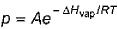Graphically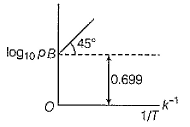Match the parameters in Column i with their values in Column II and select answer from codes given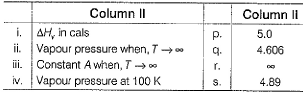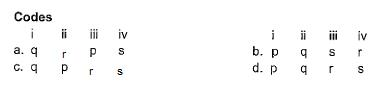Solution:
*Answer can only contain numeric values
QUESTION: 17

Direction (Q. Nos. 16 and 17) This section contains 2 questions. when worked out will result in an integer from 0 to 9 (both inclusive).

Q. The vapour pressure of benzene C6H6 at 298 K is 95 torr. After 10.00 g of benzene is injected into a 10.0 L bulb at 298 K, how many grams of benzene remain as liquid?

Solution:
*Answer can only contain numeric values
QUESTION: 18

113 L of helium gas at 1360°C and prevailing barometric pressure is passed through molten silver at 1360°C. The gas becomes saturated with silver vapour. As a result, the liquid silver loses 0.120 g in mass. What is the vapour pressure (in mm Hg) of liquid silver at 1360°C? Neglect any change in volume due to vaporisation of the silver.

Solution: